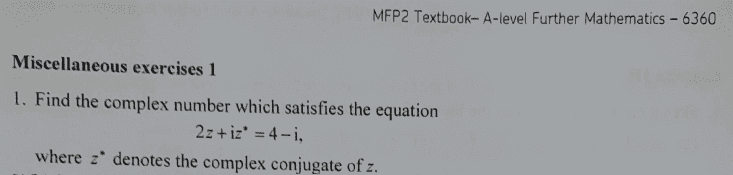# Find the complex number which satisfies the given equation

• chwala

#### chwala

Gold Member
Homework Statement
see attached
Relevant Equations
complex numbers
Find the problem here; ( i do not have the solutions...i seek alternative ways of doing the problems)ok, i let ##z=x+iy## and ##z^*= x-iy##... i ended up with the simultaneous equation;
##2x+y=4##
##x+2y=-1##

##x=1## and ##y=2##

therefore our complex number is ##z=1+2i##

Homework Statement:: see attached
Relevant Equations:: complex numbers

Find the problem here; ( i do not have the solutions...i seek alternative ways of doing the problems)

View attachment 297755

ok, i let ##z=x+iy## and ##z^*= x-iy##... i ended up
How?
with the simultaneous equation;
##2x+y=4##
##x+2y=-1##

##x=1## and ##y=2##
##1+2\cdot 2 =5 \neq -1##
therefore our complex number is ##z=1+2i##

How?

##1+2\cdot 2 =5 \neq -1##
let me check this...

Arrrgh##x=3## and ##y=-2## ...sign error on my simultaneous equation...i guess its best to solve this questions over the weekend...i rush on this due to other work...

How?
fresh from complex numbers we know that, if ##w=a+in## then ##w^* =a-in##

Last edited by a moderator:
I meant: How you ended up with these equations, not how conjugation is defined, i.e. show your steps.

I meant: How you ended up with these equations, not how conjugation is defined, i.e. show your steps.
Ooh ok...noted Fresh...